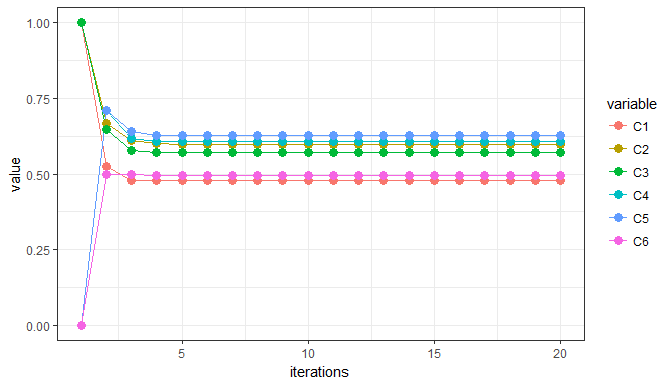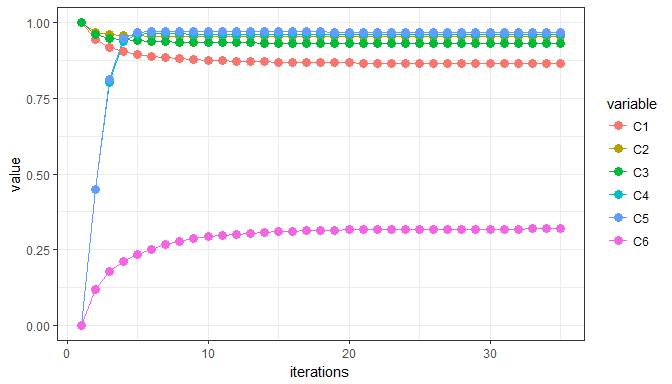# Inference of Fuzzy Cognitive Maps (FCMs)

### The fcm package

Estimates the Inference of a Fuzzy Cognitive Map. Moreover, the “fcm” package provides a selection of 3 different inference rules and 4 threshold functions in order to obtain the FCM inference. It returns a dataframe of the concepts’ values of each state after the inference procedure. FCM is proven to be capable of causal inference and is applicable to modeling complex decision problems where numerous interlinked dependent variables influence one another.

#### Fuzzy Cognitive Maps (FCMs)

Fuzzy Cognitive Map is a combination of fuzzy logic and cognitive mapping, and it is a way to represent knowledge of systems which are characterized of uncertainty and complex processes. FCMs were introduced by Kosko and since then they have gradually emerged as a powerful paradigm for knowledge representation. FCMs are ideal causal cognition tools for modeling and simulating dynamic systems.

FCMs are fuzzy signed directed graphs with feedback. Each interconnection between two concepts $$C_{i}$$ and $$C_{j}$$ has a weight, a directed edge $$w_{ij}$$, which indicates the strength of the causal relationships between concepts $$C_{i}$$ and $$C_{j}$$. The value of the weight $$w_{ij}$$ indicates how strongly the concept $$C_{i}$$ influences concept $$C_{j}$$.The time varying concept function $$C_{i}(t)$$ measures the non negative occurrence of some fuzzy event. There are three possible types of causal relationship between concept $$C_{i}$$ and concept $$C_{j}$$, according to the sign of the weight $$w_{ij}$$:

1. $$w_{ij}>0$$ indicates a positive causality between concept $$C_{i}$$ and concept $$C_{j}$$. This means that an increase/decrease in the value of concept $$C_{i}$$ leads to the increase/decrease of the value of concept $$C_{j}$$. (positive causality)
2. $$w_{ij}<0$$ indicates a negative (inverse) causality between concept $$C_{i}$$ and concept $$C_{j}$$. This means that an increase in the value of concept $$C_{i}$$ leads to a decrease of the value of concept $$C_{j}$$ and a decrease of the value of concept $$C_{i}$$ leads to an increase of the value of concept $$C_{j}$$. ( negative causality)
3. $$w_{ij}=0$$ indicates no relationship between concept $$C_{i}$$ and $$C_{j}$$. (zero causality)

#### Inference Rules

Every concept in the FCM graph has a value $$A_{i}$$ that expresses the quantity of its corresponding physical value and it is derived by the transformation of the fuzzy values assigned by the experts to numerical values. The value $$A_{i}$$ of each concept $$C_{i}$$ is calculated during each simulation step, computing the influence of other concepts to the specific concept by selecting one of the following equations (inference rules):

Inference Rule Equation
Kosko’s inference: $$A_{i}(k+1)=f\left(\sum_{j=1,j\neq1}^Nw_{ji}\times A_{j}(k)\right)$$
modified Kosko’s inference: $$A_{i}(k+1)=f\left(A_{i}(k)+\sum_{j=1,j\neq1}^Nw_{ji}\times A_{j}(k)\right)$$
Rescale inference: $$A_{i}(k+1)=f\left((2\times A_{i}(k)-1)+\sum_{j=1,j\neq1}^Nw_{ji}\times (2\times A_{j}(k)-1)\right)$$

where $$A_{i}(k+1)$$ is the value of concept $$C_{i}$$ at simulation step $$k+1$$, $$A_{j}(k)$$ is the value of concept $$C_{j}$$ at the simulation step $$k$$, $$w_{ij}$$ is the weight of the interconnection between concept $$C_{j}$$ and concept $$C_{i}$$, $$k$$ is the interaction index an every simulation step and $$f(·)$$ is the threshold (activation) function:

Threshold function Equation
Bivalent: $f(x)= \left\{\begin{array}{ll} 1 & x > 0 \\0 & x\leq 0 \\\end{array} \right.$
Trivalent: $f(x)= \left\{\begin{array}{ll} 1 & x > 0 \\0 & x=0 \\-1 & x<0 \\\end{array} \right.$
Sigmoid: $$f(x)=\frac{1}{1+e^{-\lambda\times x}}$$
Hyperbolic tangent: $$f(x)=\tanh(\lambda\times x)$$

where $$\lambda$$ is a real positive number ($$\lambda>0$$) which determines the steepness of the continuous function $$f$$ and $$x$$ is the value $$A_{i}(k)$$ on the equilibrium point. The sigmoid threshold function ensures that the calculated value of each concept will belong to the interval [0,1]. When the values of concepts can be negative and their values belong to the interval [-1,1], the following function is used: $$f(x)=tanh(x)$$. The FCM model of the system takes the initial values of concepts and weights based on experts knowledge and experience for the real system and then it is free to interact. At each step, the value $$A_{i}$$ of a concept is influenced by the values of concepts connected to it and it is updated according to the inference rule.

NOTE: Except the inference rules that mentioned above, the clamping types of these rules are determined (Kosko-clamped, modified Kosko-clamped and Rescale-clamped). In this case, the activated concepts (concept’s value equals to 1) are activated in all states in the FCM simulation until the output is stabilized.

## How is it possible to obtain the FCM inference using R?

### Step 1: Load the fcm package

library(fcm)

### Step 2: Create (or import) the weight matrix and the activation vector

The weight matrix stores the weights assigned to the pairs of concepts which are usually normalized to the interval $$[0, 1]$$ or $$[-1, +1]$$. The dimension of the weight matrix is m x m, where m denotes the number of the columns (nodes).

The activation vector contains the initial concept values which each concept is turned on or activated by making its vector element 1 or 0 or in $$[0, 1]$$. The dimension of the activation matrix is 1 x m.

NOTE: Both of these input arguments should be transformed as dataframes.

act.vec <- data.frame(1, 1, 1, 0, 0, 0)    # Create the activation vector
colnames(act.vec) <- c("C1", "C2", "C3", "C4", "C5", "C6")  # Change the column names

C1 = c(0.0, 0.0, 0.6, 0.9, 0.0, 0.0)
C2 = c(0.1, 0.0, 0.0, 0.0, 0.0, 0.0)
C3 = c(0.0, 0.7, 0.0, 0.0, 0.9, 0.0)
C4 = c(0.0, 0.0, 0.0, 0.0, 0.0, 0.9)
C5 = c(0.0, 0.0, 0.0, 0.0, 0.0, -0.9)
C6 = c(-0.3, 0.0, 0.0, 0.0, 0.0, 0.0)

w.mat <- matrix(c(C1, C2, C3, C4, C5, C6), nrow =6, ncol=6, byrow=TRUE)   # Create the weight matrix
w.mat <- as.data.frame(w.mat)    # Transform w.mat as a dataframe
colnames(w.mat) <- c("C1", "C2", "C3", "C4", "C5", "C6")
w.mat       # View the weight matrix
##     C1  C2  C3  C4  C5   C6
## 1  0.0 0.0 0.6 0.9 0.0  0.0
## 2  0.1 0.0 0.0 0.0 0.0  0.0
## 3  0.0 0.7 0.0 0.0 0.9  0.0
## 4  0.0 0.0 0.0 0.0 0.0  0.9
## 5  0.0 0.0 0.0 0.0 0.0 -0.9
## 6 -0.3 0.0 0.0 0.0 0.0  0.0

NOTE: It is possible to import a file (for instance a .csv file), typing the file path.
w.mat <- read.csv("C://type_the_file_path//name_of_file.csv")

For more information about reading .csv files, type in R console: ?read.csv.

### Step 3: Use the fcm.infer function

There are seven input arguments in the fcm.infer function:

Arguments Explanation
activation_vec The activation vector contains the initial concept values which each concept is turned on or activated by making its vector element 1 or 0 or in $$[0,1]$$
weight_mat The weight matrix stores the weights assigned to the pairs of concepts which are usually normalized to the interval $$[0, 1]$$ or $$[-1, +1]$$
iter The number of iterations is a positive number ($$iter > 0$$). Default value: $$iter = 20$$
infer Six different inference rules are available (Kosko: 'k', modified Kosko: 'mk', Rescale: 'r', Kosko-clamped: 'kc', modified Kosko-clamped: 'mkc' or Rescale-clamped: 'rc'). Default value: infer = 'k'
transform Four transformation functions (Bivalent: 'b', Trivalent: 'tr', Sigmoid: 's' or Hyperbolic tangent: 't'). Default value: transform = 's'
lambda The lambda ($$\lambda$$) parameter is a positive number, higher than zero and lower-equal to ten, $$0<\lambda\leq10$$. Default value: $$lambda = 1$$
e Epsilon ($$\epsilon$$) is a residual, describing the minimum error difference among the subsequent concepts. Its value depends on the application type. The possible $$e$$ value is determined between 0.01 and 0.000001. Default value: $$e = 0.001$$

The fcm.infer function prints a message to inform the user if the convergence has been reached or not.

• If the convergence has been reached then the converged state (fixed point) is printed:

"The concepts' values are converged in the ith state".

• If the convergence has not been reached a message informs the user that more iterations required:

"More iterations are required to reach the convergence".

#### Examples to obtain the FCM Inference

Example 1: Estimate the FCM Inference (using the default values of the function)

output1 <- fcm.infer(act.vec, w.mat)
##
##  The concepts' values are converged in the 6th state (e <= 0.001000)
##
##         C1        C2        C3        C4        C5        C6
##  0.4778365 0.5986462 0.5712033 0.6059079 0.6257586 0.4955168

Example 2: Estimate the FCM Inference (changing the default values)

The FCM Inference (fcm.infer) function is using the rescale 'r' inference rule, the sigmoid 's' transformation function, the lambda parameter equals to 2 lambda = 2 and the e parameter e = 0.0001.

output2 <- fcm.infer(act.vec, w.mat, 35, "r", "s", lambda = 2, e = 0.0001)
##
##  The concepts' values are converged in the 30th state (e <= 0.000100)
##
##         C1        C2        C3        C4        C5        C6
##  0.8654104 0.9534946 0.9309104 0.9589686 0.9684847 0.3186077
output2$values # View the concepts' values for each iteration ## C1 C2 C3 C4 C5 C6 ## 1 1.0000000 1.0000000 1.0000000 0.0000000 0.0000000 0.0000000 ## 2 0.9426758 0.9677045 0.9608343 0.4501660 0.4501660 0.1192029 ## 3 0.9179445 0.9593460 0.9481304 0.8012773 0.8114818 0.1789925 ## 4 0.9038475 0.9565677 0.9424370 0.9375972 0.9457921 0.2106909 ## 5 0.8952183 0.9554297 0.9392881 0.9609935 0.9669441 0.2338428 ## 6 0.8891841 0.9548570 0.9373607 0.9632707 0.9692077 0.2523626 ## 7 0.8845094 0.9545244 0.9360451 0.9628218 0.9692708 0.2666038 ## 8 0.8807890 0.9543063 0.9350512 0.9621493 0.9691369 0.2775191 ## 9 0.8778103 0.9541466 0.9342632 0.9615592 0.9690137 0.2859616 ## 10 0.8754189 0.9540220 0.9336277 0.9610727 0.9689135 0.2925587 ## 11 0.8734943 0.9539220 0.9331126 0.9606759 0.9688324 0.2977590 ## 12 0.8719415 0.9538409 0.9326946 0.9603529 0.9687666 0.3018871 ## 13 0.8706860 0.9537751 0.9323549 0.9600900 0.9687130 0.3051822 ## 14 0.8696690 0.9537215 0.9320786 0.9598760 0.9686694 0.3078236 ## 15 0.8688440 0.9536779 0.9318538 0.9597017 0.9686340 0.3099481 ## 16 0.8681740 0.9536423 0.9316707 0.9595596 0.9686050 0.3116613 ## 17 0.8676294 0.9536134 0.9315216 0.9594438 0.9685815 0.3130458 ## 18 0.8671865 0.9535898 0.9314000 0.9593494 0.9685623 0.3141663 ## 19 0.8668261 0.9535705 0.9313010 0.9592724 0.9685466 0.3150745 ## 20 0.8665327 0.9535549 0.9312203 0.9592096 0.9685338 0.3158112 ## 21 0.8662938 0.9535421 0.9311544 0.9591584 0.9685234 0.3164094 ## 22 0.8660993 0.9535316 0.9311008 0.9591167 0.9685149 0.3168954 ## 23 0.8659408 0.9535231 0.9310571 0.9590827 0.9685080 0.3172905 ## 24 0.8658118 0.9535162 0.9310214 0.9590550 0.9685023 0.3176117 ## 25 0.8657066 0.9535105 0.9309924 0.9590324 0.9684977 0.3178731 ## 26 0.8656210 0.9535059 0.9309687 0.9590139 0.9684940 0.3180857 ## 27 0.8655513 0.9535022 0.9309494 0.9589989 0.9684909 0.3182588 ## 28 0.8654944 0.9534991 0.9309337 0.9589867 0.9684884 0.3183997 ## 29 0.8654481 0.9534966 0.9309208 0.9589767 0.9684864 0.3185144 ## 30 0.8654104 0.9534946 0.9309104 0.9589686 0.9684847 0.3186077 ## 31 0.8653797 0.9534929 0.9309019 0.9589620 0.9684834 0.3186838 ## 32 0.8653547 0.9534916 0.9308950 0.9589566 0.9684823 0.3187457 ## 33 0.8653343 0.9534905 0.9308893 0.9589522 0.9684814 0.3187961 ## 34 0.8653177 0.9534896 0.9308847 0.9589486 0.9684807 0.3188372 ## 35 0.8653042 0.9534888 0.9308810 0.9589457 0.9684801 0.3188707 ### Step 4: Visualize the concepts’ values for each iteration For the visualization of the concepts’ values for each iteration after the inference process, it is crucial to load the libraries: reshape and ggplot2. # load the libraries library (reshape2) library (ggplot2) Visualization of Example 1: iterations <- as.numeric(rownames(output1$values))  # create a numeric vector named "iterations"
df <- data.frame(iterations, output1$values) # add "iterations" in the "output1$values" dataframe
df2 <- melt(df, id="iterations")              # transform the dataframe df into long formats
ggplot(data=df2,                              # Visualize the concepts' values
aes(x=iterations, y=value, group=variable, colour=variable)) +
theme_bw() + geom_line(size=0.7) + geom_point(size = 3)Visualization of Example 2:

iterations <- as.numeric(rownames(output2$values)) df <- data.frame(iterations, output2$values)
df2 <- melt(df, id="iterations")
ggplot(data=df2,
aes(x=iterations, y=value, group=variable, colour=variable)) +
theme_bw() + geom_line(size=0.7) + geom_point(size = 3)